# Resources tagged with: Multiplication & division

Filter by: Content type:
Age range:
Challenge level:

### There are 152 results

Broad Topics > Calculations and Numerical Methods > Multiplication & division### Ducking and Dividing

##### Age 7 to 11 Challenge Level:

Your vessel, the Starship Diophantus, has become damaged in deep space. Can you use your knowledge of times tables and some lightning reflexes to survive?### Remainders

##### Age 7 to 14 Challenge Level:

I'm thinking of a number. My number is both a multiple of 5 and a multiple of 6. What could my number be?### Missing Multipliers

##### Age 7 to 14 Challenge Level:

What is the smallest number of answers you need to reveal in order to work out the missing headers?### Factoring Factorials

##### Age 11 to 14 Challenge Level:

Find the highest power of 11 that will divide into 1000! exactly.### What Two ...?

##### Age 7 to 11 Short Challenge Level:

56 406 is the product of two consecutive numbers. What are these two numbers?### Times Right

##### Age 11 to 16 Challenge Level:

Using the digits 1, 2, 3, 4, 5, 6, 7 and 8, mulitply a two two digit numbers are multiplied to give a four digit number, so that the expression is correct. How many different solutions can you find?### Multiples Sudoku

##### Age 11 to 14 Challenge Level:

Each clue in this Sudoku is the product of the two numbers in adjacent cells.### Thirty Six Exactly

##### Age 11 to 14 Challenge Level:

The number 12 = 2^2 � 3 has 6 factors. What is the smallest natural number with exactly 36 factors?### The Remainders Game

##### Age 7 to 14 Challenge Level:

Play this game and see if you can figure out the computer's chosen number.### Eminit

##### Age 11 to 14 Challenge Level:

The number 8888...88M9999...99 is divisible by 7 and it starts with the digit 8 repeated 50 times and ends with the digit 9 repeated 50 times. What is the value of the digit M?### Powerful Factorial

##### Age 11 to 14 Challenge Level:

6! = 6 x 5 x 4 x 3 x 2 x 1. The highest power of 2 that divides exactly into 6! is 4 since (6!) / (2^4 ) = 45. What is the highest power of two that divides exactly into 100!?### Tom's Number

##### Age 7 to 11 Challenge Level:

Work out Tom's number from the answers he gives his friend. He will only answer 'yes' or 'no'.### Oh! Hidden Inside?

##### Age 11 to 14 Challenge Level:

Find the number which has 8 divisors, such that the product of the divisors is 331776.### Product Sudoku

##### Age 11 to 16 Challenge Level:

The clues for this Sudoku are the product of the numbers in adjacent squares.### Funny Factorisation

##### Age 11 to 16 Challenge Level:

Using the digits 1 to 9, the number 4396 can be written as the product of two numbers. Can you find the factors?### A First Product Sudoku

##### Age 11 to 14 Challenge Level:

Given the products of adjacent cells, can you complete this Sudoku?### Divide it Out

##### Age 7 to 11 Challenge Level:

What is the lowest number which always leaves a remainder of 1 when divided by each of the numbers from 2 to 10?### Escape from the Castle

##### Age 7 to 11 Challenge Level:

Skippy and Anna are locked in a room in a large castle. The key to that room, and all the other rooms, is a number. The numbers are locked away in a problem. Can you help them to get out?### Why 8?

##### Age 11 to 14 Challenge Level:

Choose any four consecutive even numbers. Multiply the two middle numbers together. Multiply the first and last numbers. Now subtract your second answer from the first. Try it with your own. . . .### Going Round in Circles

##### Age 11 to 14 Challenge Level:

Mathematicians are always looking for efficient methods for solving problems. How efficient can you be?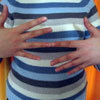### What Is the Question?

##### Age 11 to 16 Challenge Level:

These pictures and answers leave the viewer with the problem "What is the Question". Can you give the question and how the answer follows?### Repeaters

##### Age 11 to 14 Challenge Level:

Choose any 3 digits and make a 6 digit number by repeating the 3 digits in the same order (e.g. 594594). Explain why whatever digits you choose the number will always be divisible by 7, 11 and 13.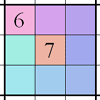### Integrated Product Sudoku

##### Age 11 to 16 Challenge Level:

This Sudoku puzzle can be solved with the help of small clue-numbers on the border lines between pairs of neighbouring squares of the grid.### Number Tracks

##### Age 7 to 11 Challenge Level:

Ben’s class were cutting up number tracks. First they cut them into twos and added up the numbers on each piece. What patterns could they see?##### Age 11 to 14 Challenge Level:

Make a set of numbers that use all the digits from 1 to 9, once and once only. Add them up. The result is divisible by 9. Add each of the digits in the new number. What is their sum? Now try some. . . .### X Marks the Spot

##### Age 11 to 14 Challenge Level:

When the number x 1 x x x is multiplied by 417 this gives the answer 9 x x x 0 5 7. Find the missing digits, each of which is represented by an "x" .### Being Resilient - Primary Number

##### Age 5 to 11 Challenge Level:

Number problems at primary level that may require resilience.### A Mixed-up Clock

##### Age 7 to 11 Challenge Level:

There is a clock-face where the numbers have become all mixed up. Can you find out where all the numbers have got to from these ten statements?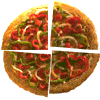### Fair Feast

##### Age 5 to 11 Challenge Level:

Here is a picnic that Petros and Michael are going to share equally. Can you tell us what each of them will have?### Double Digit

##### Age 11 to 14 Challenge Level:### Odds and Threes

##### Age 7 to 11 Challenge Level:

A game for 2 people using a pack of cards Turn over 2 cards and try to make an odd number or a multiple of 3.### Code Breaker

##### Age 7 to 11 Challenge Level:

This problem is based on a code using two different prime numbers less than 10. You'll need to multiply them together and shift the alphabet forwards by the result. Can you decipher the code?### Book Codes

##### Age 7 to 11 Challenge Level:

Look on the back of any modern book and you will find an ISBN code. Take this code and calculate this sum in the way shown. Can you see what the answers always have in common?### One O Five

##### Age 11 to 14 Challenge Level:

You can work out the number someone else is thinking of as follows. Ask a friend to think of any natural number less than 100. Then ask them to tell you the remainders when this number is divided by. . . .### What Is Ziffle?

##### Age 7 to 11 Challenge Level:

Can you work out what a ziffle is on the planet Zargon?### Largest Product

##### Age 11 to 14 Challenge Level:

Which set of numbers that add to 10 have the largest product?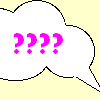### A Conversation Piece

##### Age 7 to 11 Challenge Level:

Take the number 6 469 693 230 and divide it by the first ten prime numbers and you'll find the most beautiful, most magic of all numbers. What is it?### A One in Seven Chance

##### Age 11 to 14 Challenge Level:

What is the remainder when 2^{164}is divided by 7?### Gabriel's Problem

##### Age 11 to 14 Challenge Level:

Gabriel multiplied together some numbers and then erased them. Can you figure out where each number was?### The Genie in the Jar

##### Age 11 to 14 Challenge Level:

This jar used to hold perfumed oil. It contained enough oil to fill granid silver bottles. Each bottle held enough to fill ozvik golden goblets and each goblet held enough to fill vaswik crystal. . . .### Jumping

##### Age 7 to 11 Challenge Level:

After training hard, these two children have improved their results. Can you work out the length or height of their first jumps?### Journeys on the Gattegno Tens Chart

##### Age 5 to 11

Alf describes how the Gattegno chart helped a class of 7-9 year olds gain an awareness of place value and of the inverse relationship between multiplication and division.### Surprising Split

##### Age 7 to 11 Challenge Level:

Does this 'trick' for calculating multiples of 11 always work? Why or why not?### Legs Eleven

##### Age 11 to 14 Challenge Level:

Take any four digit number. Move the first digit to the end and move the rest along. Now add your two numbers. Did you get a multiple of 11?##### Age 11 to 14 Challenge Level:

Visitors to Earth from the distant planet of Zub-Zorna were amazed when they found out that when the digits in this multiplication were reversed, the answer was the same! Find a way to explain. . . .### Square Subtraction

##### Age 7 to 11 Challenge Level:

Look at what happens when you take a number, square it and subtract your answer. What kind of number do you get? Can you prove it?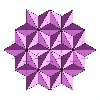### Next Number

##### Age 7 to 11 Short Challenge Level:

Find the next number in this pattern: 3, 7, 19, 55 ...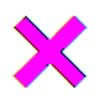### Learning Times Tables

##### Age 5 to 11 Challenge Level:

In November, Liz was interviewed for an article on a parents' website about learning times tables. Read the article here.### Sort Them Out (2)

##### Age 7 to 11 Challenge Level:

Can you each work out the number on your card? What do you notice? How could you sort the cards?### As Easy as 1,2,3

##### Age 11 to 14 Challenge Level:

When I type a sequence of letters my calculator gives the product of all the numbers in the corresponding memories. What numbers should I store so that when I type 'ONE' it returns 1, and when I type. . . .